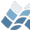NV5 GEOSPATIAL SOFTWAREEMD

EMD

## Purpose

This function estimates the empirical mode decomposition of a given
data vector.

## Category

Time series analysis

## Calling Sequence

Result = EMD( Data )

## Input

Data: A floating point vector containing the input data values.

## Keyword Parameters

QUEK: If set, the procedure test for IMFs by checking the size of
the difference between successive rounds but with a modified
comparison as adopted by Quek et alii (2003). The default is
without the modification.
SHIFTFACTOR: A floating point factor to be used in comparing
normalised squared differences between successive rounds when
testing for IMFs. The default is 0.3.
SPLINEMEAN: If set, the procedure estimates the local mean by splining
between the mean of the extrema. The default is to take the
mean of the splines through the extrema.
VERBOSE: If set, the procedure prints some output and error warnings.
The default is not set.
ZEROCROSS: If set, the procedure tests for IMFs by comparing the
number of extrema and zero crossings. The default is by
checking the size of the difference between successive rounds.

## Output

Result: Returns a floating point matrix containing the intrinsic mode
functions (IMFs). The dimensions are number of time steps by
number of IMFs.

EXTREMA.pro
VAR_TYPE.pro
ZERO_CROSS.pro

## Procedure

This function uses an iterative method to decompose a time series into
intrinsic mode functions according to Huang et alii.

## Example

Given a time series DATA.
imf = EMD( data )
Plot the data.
plot, data
Overplot the sum of the IMFs. This should be identical to DATA.
tek_color
oplot, total( imf, 2 ), color=2

## References

Huang et al, Royal Society Proceedings on Math, Physical,
and Engineering Sciences, vol. 454, no. 1971, pp. 903-995,
8 March 1998
Coughlin et al. (2003), in press.
Quek, et al. (2003), Smart Mater. Struct., 12, 447-460.

## Modification History

MatLab: Ivan Magrin-Chagnolleau (ivan@ieee.org)
Matlab: Anthony Wilson (anthony.wilson:zoo.ox.ac.uk), 2003
Written by: Daithi A. Stone (stoned@atm.ox.ac.uk), 2003-12-18